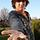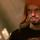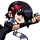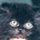## General Question# Why does Integral[(e^sqrt(x)) / sqrt(x)] NOT equal e^sqrt(x) + C? Details of how I got there inside...

Asked by HeroicZach (195) May 2nd, 2009

So I tried this with u substitution, since I think that’s how you should approach these types.

u = sqrt(x) = x^(1/2)

Set up differential equation:

du = 1/sqrt(x) dx

Substitute back into main integral:

Integral(e^u du)

Solve:

e^u + C

Substitute:

e^sqrt(x) + C

That’s my reasoning, and I think it’s OK! That was also one of the multiple choice answers, but it was one of my many questions marked wrong. What’s up?

Observing members: 0Composing members: 0The derivative of sqrt(x) isn’t 1/sqrt(x), it is .5/sqrt(x), because sqrt(x) can be rewritten as x^.5, and the chain rule applies and makes the derivative .5*x^-.5

Jayne (6776)“Great Answer” (0) Flag as…Well first of all, Jayne is correct, your du term is incorrect. That being said, I am blanking on how to actually solve this.

In case you missed it before your last question got modded, that derivative is not what Jayne posted. It’s -12sin(4x)cos^2(4x)

Ivan (13479)“Great Answer” (0) Flag as…@Ivan Well I think now that I know that du = 1/2sqrt(x) dx then I would substitute that back in and extract the 1/2 to the outside of the integral, so

Substitute back into main integral

(1/2) Integral(e^u du)

Solve:

(1/2) e^u + C

Substitute:

1/(2 * e^sqrt(x) ) + C

That is also one of the answer choices, probably the right one too (I wish my teacher actually marked the right answers for the ones I got wrong).

HeroicZach (195)“Great Answer” (0) Flag as…Wow, it’s amazing how much of this stuff I forgot. It’s been way too long

Yeah, it’s definitely 2e^sqrt(x).

u = x^1/2

du = (1/2)x^-1/2

2du = x^-1/2

substitute: integral[2du*e^u]

= 2e^u

= 2e^x^1/2

Ivan (13479)“Great Answer” (0) Flag as…@Ivan; yeah, I went back to change that, but it got snatched too quickly.

Anyway, Ivan has the right answer. No need to do substitution; just recognize that the integral, when differentiating, will be multiplied by 1/2 because of the chain rule, and must therefore be given a constant of two to cancel this. However, if you want to formalize the process with substitution, your mistake was that, if u=sqrt(x), then F’(x)=2e^u du, not (1/2)e^u du.

Jayne (6776)“Great Answer” (0) Flag as…@Jayne

Sorry to keep ninja’ing you!

Yeah, you can reason your way through this integral. If you wanted to use u-substitution, I detailed how it’s done above.

A long time ago, I got a 5 out of 5 on this test. This is fun trying to remember how to do all this stuff again. It’s all coming back.

Ivan (13479)“Great Answer” (0) Flag as……Where will you ever use this in life…just curious.

hateants (11)“Great Answer” (0) Flag as…Umm, @hateants…any career in science or engineering uses calculus constantly. None of the technology you use could exist if it were not for calculus. Hell, even historians or anthropologists use calculus to analyze changes over time, and isotopic dating of artifacts relies on mathematical tools derived from calculus. Basic stuff like this would be handled by computers, but you can’t learn to manipulate complex ideas without knowing how you got there, now can you?

Jayne (6776)“Great Answer” (1) Flag as…@hateants

If your career even is even remotely related to science, you will be using basic calculus such as this.

Ivan (13479)“Great Answer” (1) Flag as…@hateants I’ve always wondered this a bit myself. Being a student, I’m a bit cynical of the whole thing, but one day I sat after school and talked about this with my calculus teacher. It’s used just about everywhere, because calculus is used to describe lots of nature. It doesn’t make sense to me yet, but apparently knowledge of the greater meaning of calculus comes with time. I know, weird huh?

HeroicZach (195)“Great Answer” (0) Flag as…or